Next: Zero padding is the Up: FAMILIAR OPERATORS Previous: FAMILIAR OPERATORS

## Transient convolution

When the matrix has a special form, such as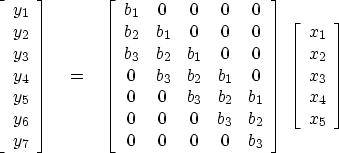(1)
then the matrix multiplication and transpose multiplication still fit easily in the same computational framework. The operation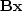convolves bt with xt, whereas the operation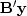crosscorrelates bt with yt. I will leave it to you to verify the pseudocode


do ix = 1, nx {
do ib = 1, nb {
iy = ib + ix - 1
if operator itself  (convolution)
y(iy) = y(iy) + b(ib)x(ix)
x(ix) = x(ix) + b(ib)y(iy)
}}


Again, notice that the bottom line'' in the program is that x and y are simply interchanged.

Equation (1) could be rewritten as(2)
which we abbreviate by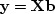.So we can choose betweenand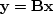.In one case the outputis dual to the filter,and in the other case the outputis dual to the input.In applications, sometimes we will solve forand sometimes for;so sometimes we will use equation (2) and sometimes (1).

The program contran()can be used with either equation (1) or equation (2), because the calling program can swap the xx and bb variables. The name contran() denotes convolution with transpose'' and with transient'' end effects.

#       Convolve and correlate (adjoint convolve).
#
real    xx(nx)                  # input signal
real    bb(nb)                  # filter      (or output crosscorrelation)
real    yy(nx+nb-1)             # filtered signal (or second input signal)
ny = nx + nb - 1                # length of filtered signal
do ib= 1, nb {
do ix= 1, nx {